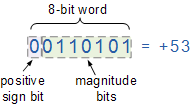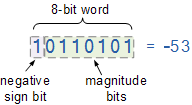×

Search anything:

# Algorithm to detect whether two numbers have opposite signs using bitwise operators

#### bitwise operation bitwise algorithm xor AlgorithmsGet this book -> Problems on Array: For Interviews and Competitive Programming

Reading time: 10 minutes | Coding time: 5 minutes

The problem at hand is to detect whether two given numbers has opposite signs using bitwise operators.

One of the simplest way to solve this problem is to check whether one number is larger than 0 and other is smaller than 0. This approach is slow as it uses comparision based operations.

Bit-wise operators are naturally fast as in programming languages, bit-wise operators take advantage of the machine architecture and runs extremely fast.

In this article, we will explore the bit-wise approach to find if two numbers have opposite signs.

Sign Bit: the sign bit is a bit in a signed number representation that indicates the sign of a number.

Let the given integers be x and y. The sign bit is 1 in negative numbers, and 0 in positive numbers.

Let us take a example of number 53Here the sign bit is 0 this means it is a positive number.Here the sign bit is 1 which means it is a negative number.

## Bitwise XOR operator( ^ )

The binary XOR (exclusive OR) operation has two inputs and one output. It is like the ADD operation which takes two arguments (two inputs) and produces one result (one output).The inputs to a binary XOR operation can only be 0 or 1 and the result can only be 0 or 1.The binary XOR operation (also known as the binary XOR function) will always produce a 1 output if either of its inputs is 1 and will produce a 0 output if both of its inputs are 0 or 1.

``````            A	 	B	 	C
0	 XOR 	0	->	0
0	 XOR 	1	->	1
1	 XOR 	0	->	1
1	 XOR 	1	->	0
``````

Determination of sign using XOR

Let the given integers are “x” and “y”. The XOR of sign bit (MSB) of “x” and “y” will be 1 if the sign bit of “x” is different from the sign bit of “y”. In other words, we can say, XOR of “x” and “y” will be negative if “x” and “y” have the opposite signs.

# Code

C Program to Detect if two integers have opposite signs using Bitwise operators.

``````
#include<stdio.h>
#include<stdbool.h>
bool
oppositeSigns ( int x , int y )
{
return ( (x ^ y) < 0 );
}
int
main()
{   int x , y;
printf ( "Enter two numbers\n" );
printf ( "Enter number 1:" );
scanf ( "%d" , &x );
printf ( "Enter number 2:" );
scanf ( "%d" , &y );

if ( oppositeSigns( x, y ) == true )
printf ( "Signs are opposite" );
else
printf ( "Signs are not opposite" );
return (0);
}
``````

The above code uses a Bitwise XOR to detect wehther two numbers have opposite signs or not.